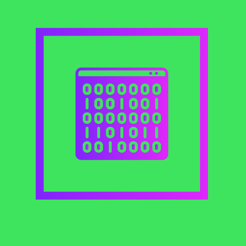## iPhone Screenshots

•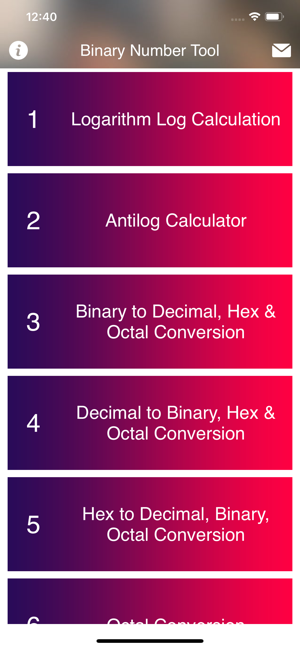•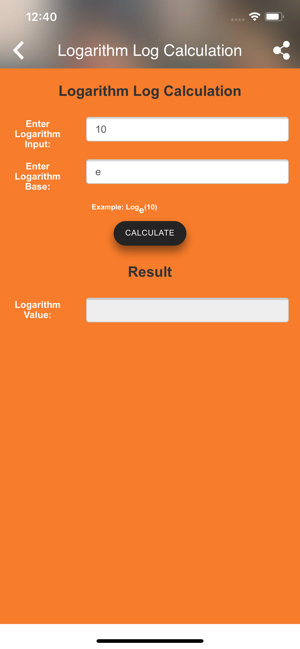•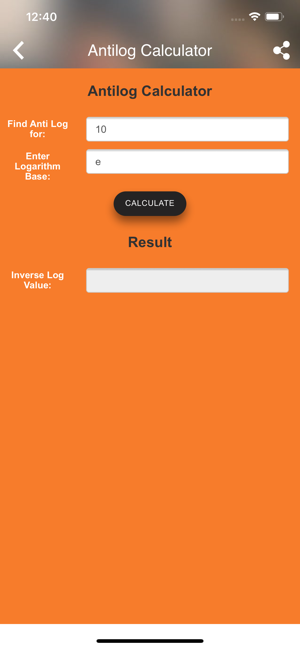•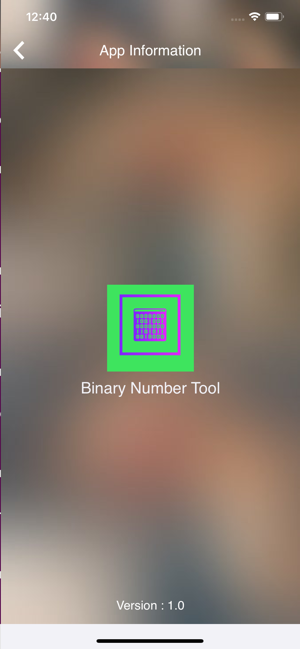## Description

Binary Number Tool supports Logarithm Log Calculation , Antilog Calculator, Binary to Decimal , Hex & Octal Conversion , Decimal to Binary , Hex & Octal Conversion , Hex to Decimal , Binary , Octal Conversion , Octal Conversion , GCD | HCF | GCF Calculation , LCM Calculation , Number to Word Conversion , LCM Calculation , Prime Number Calculation , Pythagorean Theorem Calculation , Square and Square Root Calculation , Cube and Cube Root Calculation , Fraction to Decimal Conversion , Decimal to Fraction Conversion , Exponential Power Calculation , Prime Factors Calculation , Fraction Simplification Percentage Calculation , Change Calculation , Percentage Difference Calculation,Radical Calculation,Fraction Comparison Calculation,Equivalent Fraction Calculation,ex - e Raised to Power x Calculation,Billion - Million - Crores - Lacs Conversion,Weighted Average Grade Calculation,Percentage Increase Calculation,Percentage Decrease Calculation,Divisibility Test Calculation,Arithmetic Progression Calculation,Sum of Squares & Cubes Calculation,Ascending & Descending Order For each number system there is a separate keyboard, so it is very easy and fast to use.

Binary Number Tool supports also decimal, hexadecimal, octal and binary numbers.

## Information

Seller
Bhut Rupabhai Shamjibhai
Size
18.2 MB
Category
Education
Compatibility

Requires iOS 9.0 or later. Compatible with iPhone, iPad, and iPod touch.

Languages

English

Age Rating
4+
•